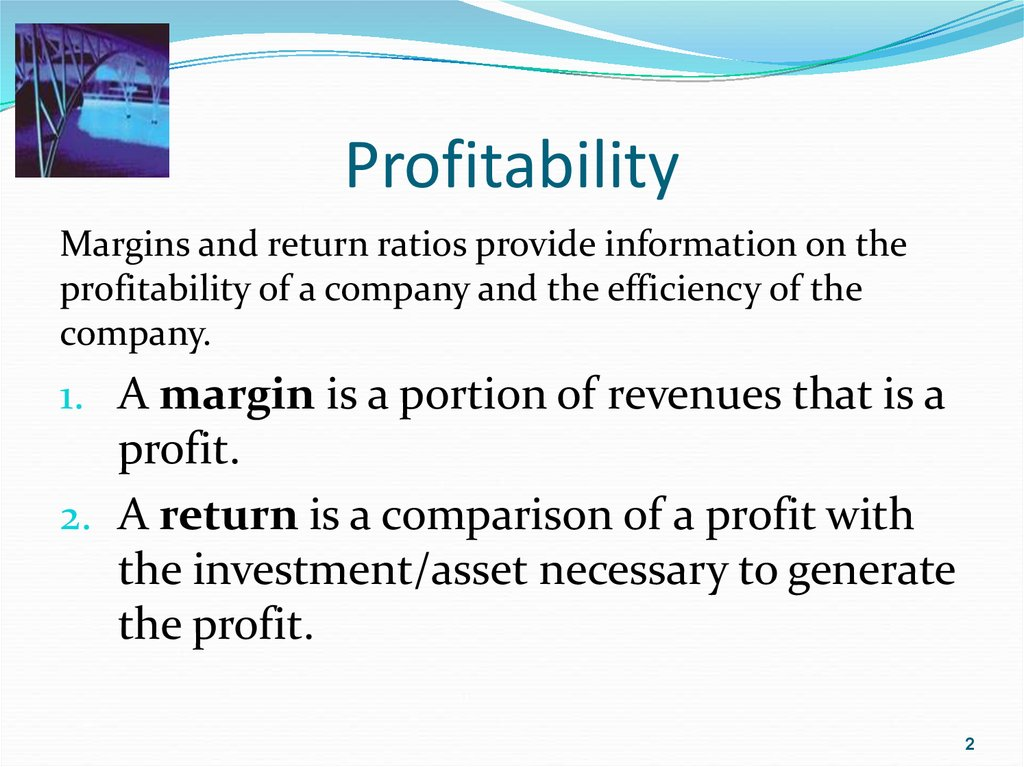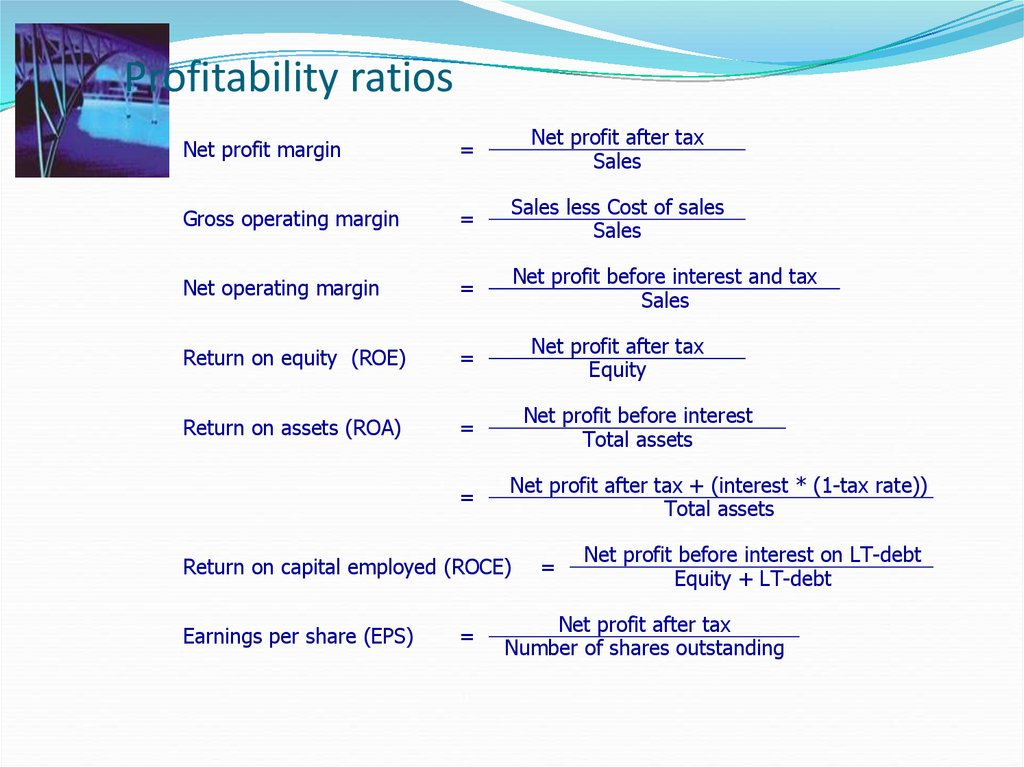# Financial Statement. Analysis

## 1.

Financial Statement
Analysis

## 2. Profitability

Margins and return ratios provide information on the
profitability of a company and the efficiency of the
company.
1. A margin is a portion of revenues that is a
profit.
2. A return is a comparison of a profit with
the investment/asset necessary to generate
the profit.
2

## 3. Profitability ratios

Net profit margin
=
Net profit after tax
Sales
Gross operating margin
=
Sales less Cost of sales
Sales
Net operating margin
=
Net profit before interest and tax
Sales
Return on equity (ROE)
=
Return on assets (ROA)
=
=
Net profit after tax
Equity
Net profit before interest
Total assets
Net profit after tax + (interest * (1-tax rate))
Total assets
Return on capital employed (ROCE)
Earnings per share (EPS)
=
=
Net profit before interest on LT-debt
Equity + LT-debt
Net profit after tax
Number of shares outstanding

4

## 5.

Earnings, (or net income,) are simply revenues minus
costs. They are an accounting measure of profits.
Earnings would not be a good measure of economic profits
given that the financial statements are subject to accounting
rules.
calculation subtracts debt interest payments and taxes owed.
Earnings Before Interest and Taxes (EBIT) is also an
important measure of profit. It includes payments that go to
debt holders and the tax authority.

## 6.

Retained earnings
Retained earnings are the earnings re-invested into the
firm:
Retained earnings = earnings - dividends
The balance sheet can grow in one of three ways:
1. Internally, through retained earnings.
2. Externally by issuing new equity.
3. Externally by issuing new debt.

## 7.

Measuring profit
Return on equity (ROE) uses accounting values: earnings
divided by book value of equity.
ROE will not be the same as the firms stock return over
the period.
Given that ROE uses accounting earnings as the profit
measure, it is sensitive to the manipulations discussed
above.
Earnings are measured over a period of time, (ie. year,)
whereas the book value of equity on the balance sheet
is at a specific point of time.

## 8.

Return on assets
Return on assets (ROA) is another important measure
of portability.
Again, ROA uses earnings to measure profit, but
divides by the firm's book value.
ROA is insensitive to the firm's financing decision.
Thus, it is a measure of operating portability.

## 9. AAA’s Balance Sheet (Asset Side)

AAA’s Balance Sheet (thousands) Dec. 31, 2015 a
Cash and C.E.
\$ 90 a. How the firm stands on a
specific date.
Account. Receivables.c
394
Inventories
696 b. What AAA owned.
Prepaid Expenses d
5 c. Amounts owed by
customers.
Accumulated Tax Prepay
10
d. Future expense items
e
Current Assets \$1,195
f
Fixed Assets (@Cost) 1030
e. Cash/likely convertible to
g
Less: Acc. Depr.
(329)
cash within 1 year.
Net Fix. Assets \$ 701
f. Original amount paid.
Investment, LT
50 g. Acc. deductions for wear
Other Assets, LT
223
and tear.
Total Assets b \$2,169

## 10. AAA’s Balance Sheet (Liability Side)

AAA Balance Sheet (thousands) Dec. 31, 2015
Notes Payable
\$ 290 a. Note, Assets = Liabilities +
Account Payablesc
94
Equity.
Accrued Taxes d
16 b. What AAA owed and
Other Accrued Liab. d
100
ownership position.
Current Liab. e \$ 500
c. Owed to suppliers for
Long-Term Debt f
530
goods and services.
Shareholders’ Equity
d. Unpaid wages, salaries,
g
Com. Stock (\$1 par) 200 Add Pd
etc.
in Capital g
729
e. Debts payable < 1 year.
h
Retained Earnings
210
f. Debts payable > 1 year.
Total Equity
\$1,139
g. Original investment.
a,b
Total Liab/Equity \$2,169
h. Earnings reinvested.

## 11. AAA’s Income Statement

AAA Profit and Losses Statement (in thousands) for Year
Ending December 31, 2015a
Net Sales
\$ 2,211
a. Measures profitability over
Cost of Goods Sold b 1,599
a time period.
Gross Profit
\$ 612
SG&A Expenses c
402
from customers.
EBITd
\$ 210
Interest Expensee
59
salaries, etc.
EBT f
\$ 151
d. Operating income.
Income Taxes
60
e. Cost of borrowed funds.
EATg
\$
91
f. Taxable income.
g. Amount earned for
Cash Dividends
38
shareholders.
Increase in RE
\$
53

## 12. Profitability Ratios

Income Statement /
Balance Sheet
Ratios
Profitability Ratios
Indicates the efficiency of
operations and firm pricing
policies.
Gross Profit Margin
Gross Profit
Net Sales
31, 2007
\$612 = .277
\$2,211

## 13. Profitability Ratio Comparisons

Gross Profit Margin
Year
2007
2006
2005
BW
27.7%
28.7
31.3
Industry
31.1%
30.8
27.6
BW has a weak Gross Profit Margin.

## 14. Gross Profit Margin -- Trend Analysis Comparison

Gross Profit Margin -Trend Analysis Comparison
Trend Analysis of Gross Profit Margin
Ratio Value (%)
35,0
32,5
30,0
BW
Industry
27,5
25,0
2005
2006
Analysis Year
2007

## 15. Profitability Ratios

Income Statement /
Balance Sheet
Ratios
Profitability Ratios
Indicates the firm’s
profitability after taking
account of all expenses and
income taxes.
Net Profit Margin
Net Profit after Taxes
Net Sales
31, 2007
\$91 = .041
\$2,211

## 16. Profitability Ratio Comparisons

Net Profit Margin
Year
2007
2006
2005
BW
4.1%
4.9
9.0
Industry
8.2%
8.1
7.6
BW has a poor Net Profit Margin.

## 17. Net Profit Margin -- Trend Analysis Comparison

Net Profit Margin -Trend Analysis Comparison
Trend Analysis of Net Profit Margin
Ratio Value (%)
10
9
8
7
BW
Industry
6
5
4
2005
2006
Analysis Year
2007

18

## 19. Profitability Ratios

Income Statement /
Balance Sheet
Ratios
Profitability Ratios
Indicates the profitability on
the assets of the firm (after
all expenses and taxes).
Return on Assets
Net Income after Taxes
Total Assets
31, 2007
\$91 = .042
\$2,160

## 20. Profitability Ratio Comparisons

Return on Investment
Year
2007
2006
2005
BW
4.2%
5.0
9.1
Industry
9.8%
9.1
10.8
BW has a poor Return on Investment.

## 21. Return on Investment – Trend Analysis Comparison

Trend Analysis of Return on Investment
Ratio Value (%)
12
10
8
BW
Industry
6
4
2005
2006
Analysis Year
2007

## 22. Profitability Ratios

Return on Equity
Income Statement /
Balance Sheet
Ratios
Profitability Ratios
Net Income after Taxes
Shareholders’ Equity
31, 2007
Indicates the profitability to
the shareholders of the firm
(after all expenses and taxes).
\$91 = .08
\$1,139

## 23. Profitability Ratio Comparisons

Return on Equity
Year
2007
2006
2005
BW
8.0%
9.4
16.6
Industry
17.9%
17.2
20.4
BW has a poor Return on Equity.

## 24. Return on Equity -- Trend Analysis Comparison

Return on Equity -Trend Analysis Comparison
Trend Analysis of Return on Equity
Ratio Value (%)
21,0
17,5
14,0
BW
Industry
10,5
7,0
2005
2006
Analysis Year
2007

## 25. The DuPont Formulas

Return on Equity
The DuPont formula uses the
relationship among financial
Total Asset
statement accounts to decompose Net Profit
Margin
Turnover
a return into components.
Three-factor DuPont for the return
on equity:
Operating Profit
Total asset turnover
Margin
Financial leverage
Net profit margin
Five-factor DuPont for the return
on equity:
Effect of Nonoperating
Items
Total asset turnover
Financial leverage
Operating profit margin
Effect of nonoperating items
Tax
Effect
Tax effect
Financial
Leverage
25

26

## 27. Example: The DuPont Formula

Suppose that an analyst has noticed that the return on equity of
the D Company has declined from FY2012 to FY2013. Using
the DuPont formula, explain the source of this decline.
(millions)
2013
2012
Revenues
Earnings before interest and taxes
Interest expense
Taxes
Total assets
Shareholders’ equity
\$1,000
\$900
\$400
\$380
\$30
\$30
\$100
\$90
\$2,000
\$2,000
\$1,250
\$1,000
27

## 28. Example: the DuPont Formula

2013
2012
Return on equity
Return on assets
0.20
0.13
0.22
0.11
Financial leverage
Total asset turnover
Net profit margin
Operating profit margin
1.60
0.50
0.25
0.40
2.00
0.45
0.24
0.42
Effect of non-operating items
Tax effect
0.83
0.76
0.82
0.71
28

29

30

## 31. Example: Shareholder ratios

Calculate the book value per share, P/E, dividends per
share, dividend payout, and plowback ratio based on the
following financial information:
Book value of equity
\$100 million
Market value of equity
\$500 million
Net income
\$30 million
Dividends
\$12 million
Number of shares
100 million
31

## 32. Example: Shareholder Ratios

Book value per share
\$1.00 There is \$1 of equity, per the books, for every
share of stock.
P/E
16.67 The market price of the stock is 16.67 times
earnings per share.
Dividends per share
\$0.12 The dividends paid per share of stock.
Dividend payout ratio
40% The proportion of earnings paid out in the
form of dividends.
Plowback ratio
60% The proportion of earnings retained by the
company.
32

## 33. Effective Use of Ratio Analysis

In addition to ratios, an analyst should describe the
company (e.g., line of business, major products, major
suppliers), industry information, and major factors or
influences.
Effective use of ratios requires looking at ratios
Over time.
Compared with other companies in the same line of
In the context of major events in the company (for
example, mergers or divestitures), accounting changes,
and changes in the company’s product mix.
33

## 34. Summary

Financial ratio analysis and common-size analysis help gauge the
financial performance and condition of a company through an
examination of relationships among these many financial items.
A thorough financial analysis of a company requires examining
its efficiency in putting its assets to work, its liquidity position,
its solvency, and its profitability.
We can use the tools of common-size analysis and financial ratio
analysis, including the DuPont model, to help understand where
a company has been.
We then use relationships among financial statement accounts
in pro forma analysis, forecasting the company’s income
statements and balance sheets for future periods, to see how the
company’s performance is likely to evolve.
34